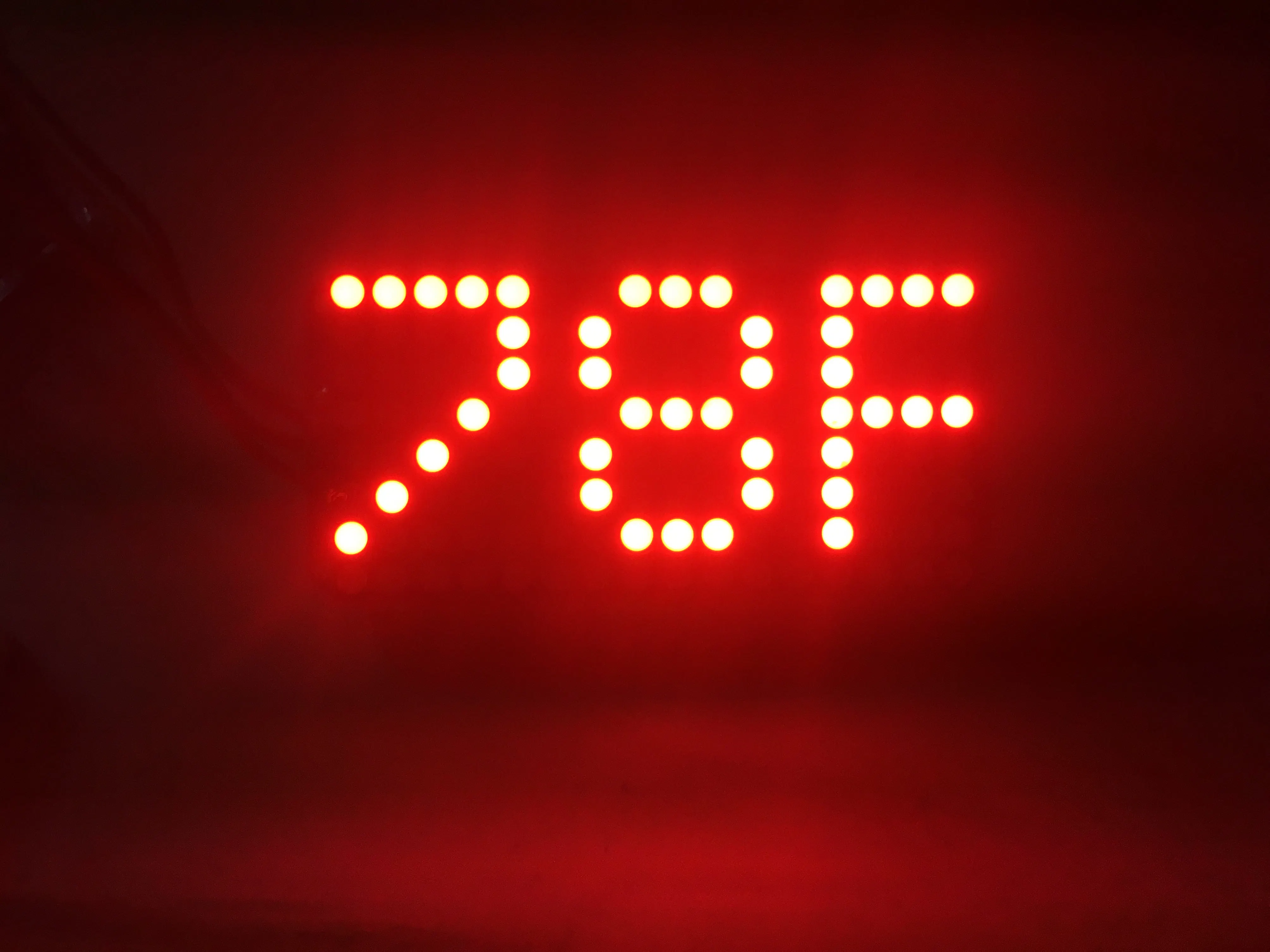Project tutorial# Periodic Temperature Reporter

This small and attractive device periodically displays an attention-catching animation followed by the current temperature in C° and F°.

• 2,123 views
• 9 respects

## Apps and online services

This project uses a couple of components to periodically display an attention-catching design followed by the current temperature in Celcius and Fahrenheit (the designs and temperatures slide in from 4 different angles). These intervals can easily be changed along with the design. The code that is used to run this project has been organized into multiple tabs with comments when appropriate.

The project uses a TMP36 to determine the current temperature, which is then converted to Celcius and Fahrenheit by the board. To catch a persons attention a quick design is displayed on a 16x8 LED Matrix from one of the four sides of the Matrix, this is then followed by the temperature in Celcius and Fahrenheit from the same side.

This is a fun and simple project that does not take long to make and has a lot variety of possible implementations.

Photo of schematics:

End expectation:

## Code

##### TemperatureReporter.inoArduino
Here there are seven different classes that work together to create the effect.
```#include <Adafruit_GFX.h>
#include "variables.h"

//TMP36 Pin Variables
int sensorPin = 0;
void setup() {
Serial.begin(9600); // matching response speed between the arduino and the computer
Serial.println("minute by minute temperature sensor");
matrix.begin(0x70);  // passes in the address
}

void loop() {
matrix.setTextSize(1); // sets text size
matrix.setTextWrap(false);  // makes the text scroll nicely
matrix.setTextColor(LED_ON);
matrix.setRotation(1); // defines the texts orientation

sense();
left();
delay(60000);
sense();
top();
delay(60000);
sense();
right();
delay(60000);
sense();
bottom();
delay(60000);
}
```
##### sense.inoArduino
```void sense() {

// converts the reading to volts, for 3.3v arduino use 3.3
voltage = reading * 5.0 / 1024.0;

// prints out the voltage
Serial.print(voltage); Serial.println(" volts");

// converts to Celcius
temperatureC = (voltage - 0.5) * 100 ;
Serial.print(temperatureC); Serial.println(" degrees C"); // prints Celcius

// converts to Fahrenheit
temperatureF = (temperatureC * 9.0 / 5.0) + 32.0;
Serial.print(temperatureF); Serial.println(" degrees F"); // prints Fahrenheit
}
```
##### top.inoArduino
```void top() {
// scrolls from top to the bottom
a = -7;
for (x=0; x<=14; x++) {
matrix.clear();
matrix.setCursor(0,a);
matrix.print("!!!");
matrix.writeDisplay();
matrix.setCursor(-1,a);
matrix.print("!!!");
matrix.writeDisplay();
delay(100);
a += 1;
b += 1;
}

for (x=-8; x<=0; x++) {
matrix.clear();
matrix.setCursor(0,x);
matrix.print(temperatureF);
delay(50);
matrix.print("F ");
delay(50);
matrix.writeDisplay();
delay(50);
}
delay(1000);

for (x=-8; x<=0; x++) {
matrix.clear();
matrix.setCursor(0,x);
matrix.print(temperatureC);
delay(30);
matrix.print("C");
delay(30);
matrix.writeDisplay();
delay(30);
}
delay(1000);
matrix.clear();
matrix.writeDisplay();
}
```
##### bottom.inoArduino
```void bottom() {
// scrolls from bottom to the top
a = 7;
b = 8;
for (x=0; x<=11; x++) {
matrix.clear();
matrix.setCursor(0,a);
matrix.print("^^^");
matrix.writeDisplay();
matrix.setCursor(0,b);
matrix.print("^^^");
matrix.writeDisplay();
delay(100);
a -= 1;
b -= 1;
}

for (x=8; x>=0; x--) {
matrix.clear();
matrix.setCursor(0,x);
matrix.print(temperatureF);
delay(50);
matrix.print("F ");
delay(50);
matrix.writeDisplay();
delay(50);
}
delay(1000);

for (x=8; x>=0; x--) {
matrix.clear();
matrix.setCursor(0,x);
matrix.print(temperatureC);
delay(30);
matrix.print("C");
delay(30);
matrix.writeDisplay();
delay(30);
}
delay(1000);
matrix.clear();
matrix.writeDisplay();
}
```
##### left.inoArduino
```void left() {
// scrolls from left to right
a = -23;
b = -24;
for (x=-20; x<=0; x++) {
matrix.clear();
matrix.setCursor(a,0);
matrix.print(">>>>");
matrix.writeDisplay();
matrix.setCursor(b,0);
matrix.print(">>>>");
matrix.writeDisplay();
delay(100);
a += 2;
b += 2;
}

for (x=-16; x<=0; x++) {
matrix.clear();
matrix.setCursor(x,0);
matrix.print(temperatureF);
delay(30);
matrix.print("F ");
delay(30);
matrix.writeDisplay();
delay(30);
}
delay(1000);

for (x=-16; x<=0; x++) {
matrix.clear();
matrix.setCursor(x,0);
matrix.print(temperatureC);
delay(30);
matrix.print("C");
delay(30);
matrix.writeDisplay();
delay(30);
}
delay(1000);
matrix.clear();
matrix.writeDisplay();
}
```
##### right.inoArduino
```void right() {
// scrolls from right to left
a = 17;
b = 18;
for (x=0; x<=21; x++) {
matrix.clear();
matrix.setCursor(a,0);
matrix.print("<<<<");
matrix.writeDisplay();
matrix.setCursor(b,0);
matrix.print("<<<<");
matrix.writeDisplay();
delay(100);
a -= 2;
b -= 2;
}

for (x=16; x>=0; x--) {
matrix.clear();
matrix.setCursor(x,0);
matrix.print(temperatureF);
delay(30);
matrix.print("F ");
delay(30);
matrix.writeDisplay();
delay(30);
}
delay(1000);

for (x=16; x>=0; x--) {
matrix.clear();
matrix.setCursor(x,0);
matrix.print(temperatureC);
delay(30);
matrix.print("C");
delay(30);
matrix.writeDisplay();
delay(30);
}
delay(1000);
matrix.clear();
matrix.writeDisplay();
}
```
##### variables.hArduino
```  int a;
int b;
float voltage;
int temperatureC;
int temperatureF;
int x;
```

## Schematics

(picture of schematics can also be seen above the story)
The design shows all the wires that are used and where they go. In this project I used and Ada Fruit 16/8 LED Matrix that did not show up in the program so I improvised by drawing wire to notes that had written the place where they needed to be connected on the matrix.
temp_reporter_design_Be3qz1vnde.fzz

October 17, 2019

#### Members who respect this project

See similar projects
you might like

#### Temperature and Humidity Logger Per Hour

Project tutorial by lefteris1993

• 3,233 views
• 13 respects

#### Temperature sensor

Project tutorial by Grant

• 26,352 views
• 44 respects

#### DHT11 Humidity + Temperature Sensor with 16x2 LCD display

Project showcase by onatto22

• 28,428 views
• 44 respects

#### Beautifully Finished Humidity and Temperature Sensor

Project tutorial by Wicked Makers

• 20,934 views
• 150 respects

#### Portable Temperature Station

Project tutorial by Isaac100

• 12,386 views